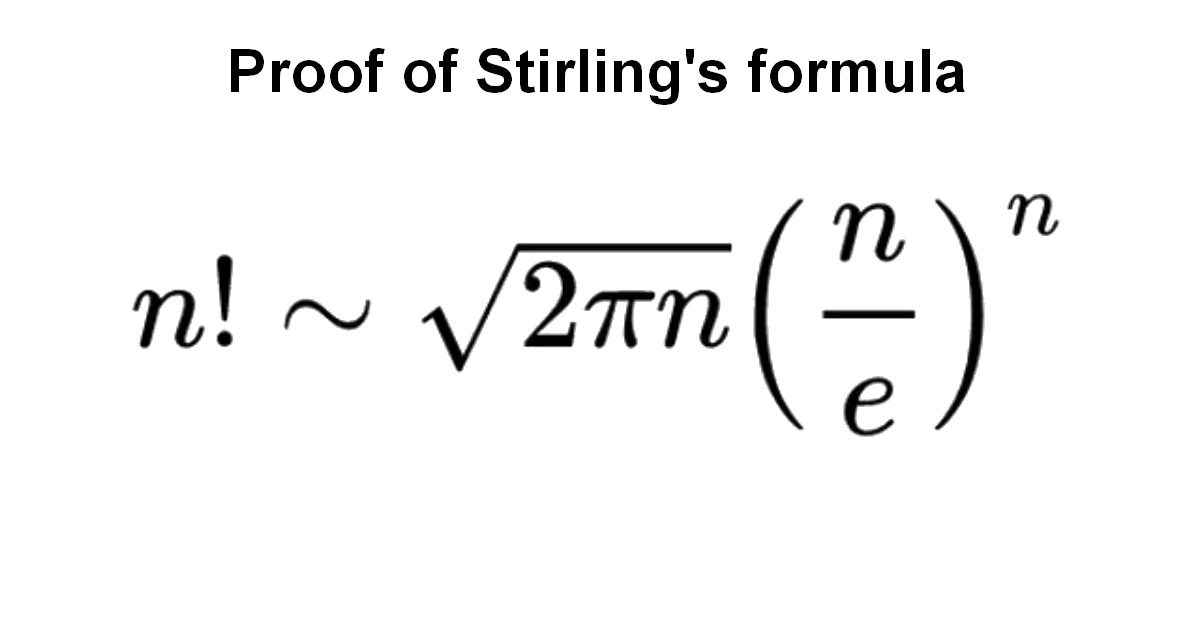## Prove Stirling's formula

Home -> Solved problems -> Stirling’s formula### Solution

Stirling’s approximation is an important approximation for factorials. It is leading to accurate results even for small values of $$n$$. It is named after James Stirling, though it was first stated by Abraham de Moivre.
First, we know that $$n!=1\cdot2\cdot\cdot\cdot n$$. We take the $$\log$$ of $$n!$$ we get $\log(n!)=\log(1)+\log(2)+\cdot\cdot\cdot+\log(n)$ The $$\log$$ function is increasing on $$(0,\infty)$$, we get $\int_{n-1}^{n}\log(x)\text{d}x<\log(n)<\int_{n}^{n+1}\log(x)\text{d}x$ for $$n\geq1$$. Let’s add the above inequalities with $$n=1,2,\cdot\cdot\cdot,N$$, we get $\int_{0}^{1}\log(x)\text{d}x<\log(1)<\int_{1}^{2}\log(x)\text{d}x$$+$$\int_{1}^{2}\log(x)\text{d}x<\log(2)<\int_{2}^{3}\log(x)\text{d}x$$+$$\cdot$$\cdot$$\cdot$$+$$\int_{N-1}^{N}\log(x)\text{d}x<\log(N)<\int_{N}^{N+1}\log(x)\text{d}x$ thus, $\int_{0}^{N}\log(x)\text{d}x<\log(1)+\log(2)+\cdot\cdot\cdot+\log(N)<\int_{1}^{N+1}\log(x)\text{d}x$ then, $\int_{0}^{N}\log(x)\text{d}x<\log(N!)<\int_{1}^{N+1}\log(x)\text{d}x$ The first integral is improper, it is not difficult to show it is convergent, we use the anti-derivative of the function $$x\rightarrow\log(x)$$ being $$x\rightarrow x\log x-x$$ and knowing that $$\lim_{x \rightarrow 0}x\log x-x=0$$ then the integral is convergent. Now let’s continue we get, $n\log(n)-n<\log(n!)<(n+1)\log(n+1)-n$ Next, let $d_{n}=\log(n!)-(n+\frac{1}{2})\log(n)+n$ Thus, $d_{n}-d_{n+1}=\log(n!)-(n+\frac{1}{2})\log(n)+n-\log((n+1)!)+(n+1+\frac{1}{2})\log(n+1)-n-1$$=\log(n!)-(n+\frac{1}{2})\log(n)+n-\log(n+1)-\log(n!)+(n+\frac{1}{2})\log(n+1)+\log(n+1)-n-1$$=(n+\frac{1}{2})\log(n+1)-(n+\frac{1}{2})\log(n)-1$$=(n+\frac{1}{2})\log(\frac{n+1}{n})-1$ With easy algebraic manipulation, we get $\frac{n+1}{n}=\frac{1+\frac{1}{2n+1}}{1-\frac{1}{2n+1}}$ The Maclaurin expansion of $$\log(x+1)$$ is $\log(x+1)=x-\frac{x^{2}}{2}+\frac{x^{3}}{3}-\frac{x^{4}}{4}+\frac{x^{5}}{5}+\cdot\cdot\cdot$
for $$-1<x\leq1$$. Now let’s find the Maclaurin expansion of $$\log(1-x)$$, to do that we replace $$x$$ by $$-x$$ in the previous expansion so we get $\log(1-x)=-x-\frac{x^{2}}{2}-\frac{x^{3}}{3}-\frac{x^{4}}{4}-\frac{x^{5}}{5}-\cdot\cdot\cdot$ for $$-1\leq x<1$$. Next let’s substract the second expansion from the first one for $$-1<x<1$$, we get $\log(1+x)-\log(1-x)=x-\frac{x^{2}}{2}+\frac{x^{3}}{3}-\frac{x^{4}}{4}+\frac{x^{5}}{5}+\cdot\cdot\cdot-(-x-\frac{x^{2}}{2}-\frac{x^{3}}{3}-\frac{x^{4}}{4}-\frac{x^{5}}{5}-\cdot\cdot\cdot)$$=x-\frac{x^{2}}{2}+\frac{x^{3}}{3}-\frac{x^{4}}{4}+\frac{x^{5}}{5}+\cdot\cdot\cdot+x+\frac{x^{2}}{2}+\frac{x^{3}}{3}+\frac{x^{4}}{4}+\frac{x^{5}}{5}+\cdot\cdot\cdot$$=2x+\frac{2}{3}x^{3}+\frac{2}{5}x^{5}+\cdot\cdot\cdot$ thus $\frac{1}{2}\log(\frac{1+x}{1-x})=x+\frac{1}{3}x^{3}+\frac{1}{5}x^{5}+\cdot\cdot\cdot$ For $$n\geq1$$ we have $$0<\frac{1}{2n+1}\leq\frac{1}{3}$$ therefore for $$x=\frac{1}{2n+1}$$, we get $\frac{1}{2}\log(\frac{1+\frac{1}{2n+1}}{1-\frac{1}{2n+1}})=\frac{1}{2n+1}+\frac{1}{3}\frac{1}{(2n+1)^{3}}+\frac{1}{5}\frac{1}{(2n+1)^{5}}+\cdot\cdot\cdot$ multiplication by $$2n+1$$, we get $\frac{1}{2}(2n+1)\log(\frac{1+\frac{1}{2n+1}}{1-\frac{1}{2n+1}})=1+\frac{1}{3}\frac{1}{(2n+1)^{2}}+\frac{1}{5}\frac{1}{(2n+1)^{4}}+\cdot\cdot\cdot$ substraction by $$1$$ $\frac{1}{2}(2n+1)\log(\frac{1+\frac{1}{2n+1}}{1-\frac{1}{2n+1}})-1=\frac{1}{3}\frac{1}{(2n+1)^{2}}+\frac{1}{5}\frac{1}{(2n+1)^{4}}+\cdot\cdot\cdot$ therefore $d_{n}-d_{n+1}=\frac{1}{3}\frac{1}{(2n+1)^{2}}+\frac{1}{5}\frac{1}{(2n+1)^{4}}+\cdot\cdot\cdot$ This implies $0<d_{n}-d_{n+1}<\frac{1}{3}(\frac{1}{(2n+1)^{2}}+\frac{1}{(2n+1)^{4}}+\cdot\cdot\cdot)$ We recognize a geometric series, therefore we have $0<d_{n}-d_{n+1}<\frac{1}{3}\frac{1}{(2n+1)^{2}-1}=\frac{1}{12}(\frac{1}{n}-\frac{1}{n+1})$ we get also $d_{n}-\frac{1}{12n}-(d_{n+1}-\frac{1}{12(n+1)})<0$ From the two previous inequalities we get  $$1.$$ The sequence $$\left\{d_{n}\right\}$$ is decreasing  $$2.$$ The sequence $$\left\{d_{n}-\frac{1}{12n}\right\}$$ is increasing  We add that $$\lim_{n \rightarrow \infty}d_{n}-(d_{n}-\frac{1}{12n})=\lim_{n \rightarrow \infty}\frac{1}{12n}=0$$, therefore $$\left\{d_{n}\right\}$$ and $$\left\{d_{n}-\frac{1}{12n}\right\}$$ are adjacent sequences.  This implies that $$\left\{d_{n}\right\}$$ converges to a number $$C$$ with $\lim_{n \rightarrow \infty}d_{n}=\lim_{n \rightarrow \infty}d_{n}-\frac{1}{12n}=C$ and that $$C\geq d_{1}-\frac{1}{12}=1-\frac{1}{12}=\frac{11}{12}$$. Taking the exponential of $$d_{n}$$ we get $e^{d_{n}}=e^{\log(n!)-(n+\frac{1}{2})\log(n)+n}=\frac{n!}{n^{(n+\frac{1}{2})}e^{-n}}$ therefore $\lim_{n \rightarrow \infty}\frac{n!}{n^{(n+\frac{1}{2})}e^{-n}}=e^{C}$ The last step is to show that $$e^{C}=\sqrt{2\pi}$$. This will be done using Wallis product $\lim_{n \rightarrow \infty}\frac{2\cdot2\cdot4\cdot4\cdot6\cdot6\cdot\cdot\cdot(2n)(2n)}{1\cdot1\cdot3\cdot3\cdot5\cdot5\cdot\cdot\cdot(2n-1)(2n-1)(2n+1)}=\frac{\pi}{2}$ Rewriting it, we get $\frac{2\cdot4\cdot6\cdot\cdot\cdot(2n)}{1\cdot3\cdot5\cdot\cdot\cdot(2n-1)\sqrt{2n}}\sim\sqrt{\frac{\pi}{2}}$ we get $\frac{(2^{n}n!)^{2}}{(2n)!}\frac{1}{\sqrt{2n}}\sim\sqrt{\frac{\pi}{2}}$ Using the approximation $n!\sim n^{(n+\frac{1}{2})}e^{-n}e^{C}$ we get $\frac{2^{2n}(n^{2n+1}e^{-2n}e^{2C})}{(2n)^{(2n+\frac{1}{2})}e^{-2n}e^{C}}\frac{1}{\sqrt{2n}}\sim\sqrt{\frac{\pi}{2}}$ Easy algebra gives $e^{C}\sim\sqrt{2\pi}$ We are dealing with constants, thus $$e^{C}=\sqrt{2\pi}$$  Finally $\huge n!\sim\sqrt{2\pi n}(\frac{n}{e})^{n}$
Home -> Solved problems -> Stirling’s formula

#### ↓ Scroll down for more maths problems↓

How far apart are the poles ?
Determine the square's side $$x$$
Find the volume of the interior of the kiln

#### ↓ ↓

Solve the equation for real values of $$x$$
Find the equation of the curve formed by a cable suspended between two points at the same height

#### ↓ ↓

Error to avoid that leads to:
Calculate the sum of areas of the three squares

#### ↓ ↓

What values of $$x$$ satisfy this inequality
Prove that the function $$f(x)=\frac{x^{3}+2 x^{2}+3 x+4}{x}$$ has a curvilinear asymptote $$y=x^{2}+2 x+3$$
Why does the number $$98$$ disappear when writing the decimal expansion of $$\frac{1}{9801}$$ ?
Only one in 1000 can solve this math problem

#### ↓ ↓

Find the limit of width and height ratio
Is $$\pi$$ an irrational number ?
Solve for $$x \in \mathbb{R}$$

#### ↓ ↓

Prove that $$e$$ is an irrational number
Solve the equation for $$x \in \mathbb{R}$$

#### ↓ ↓

Calculate the following limit
Calculate the following limit
Find the derivative of $$y$$ with respect to $$x$$

#### ↓ ↓

Prove Wallis Product Using Integration
Calculate the volume of Torus using cylindrical shells
Find the derivative of exponential $$x$$ from first principles

#### ↓ ↓

Find the volume of the square pyramid as a function of $$a$$ and $$H$$ by slicing method.
Prove that $\lim_{x \rightarrow 0}\frac{\sin x}{x}=1$
Calculate the half derivative of $$x$$

#### ↓ ↓

Find out what is a discriminant of a quadratic equation.
Calculate the rectangle's area
Determine the square's side $$x$$

#### ↓ ↓

Wonderful math fact: 12542 x 11 = 137962
What is the new distance between the two circles ?
Solve the equation for $$x\epsilon\mathbb{R}$$

#### ↓ ↓

Calculate the area of the Squid Game diagram blue part

#### ↓ ↓

Find the length of the black segment
Prove that pi is less than 22/7

#### ↓ ↓

What is the weight of all animals ?
Determine the length $$x$$ of the blue segment
How many triangles does the figure contain ?

#### ↓ ↓

if we draw an infinite number of circles packed in a square using the method shown below, will the sum of circles areas approach the square's area?
What is the value of the following infinite product?
Which object weighs the same as the four squares?
What is $$(-1)^{\pi}$$ equal to?

#### ↓ ↓

Calculate the integral $$\int_{0}^{1}(-1)^{x} d x$$

#### ↓ ↓

Find the general term of the sequence
Find the radius of the blue circles
Determine the area of the green square

#### ↓ ↓

What is the radius of the smallest circle ?

#### ↓ ↓

Find the Cartesian equation of the surface
Home -> Solved problems -> Stirling’s formula